### It's a Trap!Hover over the thumbnail for a full-size version.

Author Life247 ackbar admiral author:life247 collab inspired n-art rated 2010-09-20 2011-01-16 4 by 14 people. \$It's a Trap!#Life247#inspired#00000000000000000000000000000000000000000000000000000000000000000000000000000000000000000000000000000000000000000000000000000000000000000000000000000000000000000000000000000000000000000000000000000000000000000000000000000000000000000000000000000000000000000000000000000000000000000000000000000000000000000000000000000000000000000000000000000000000000000000000000000000000000000000000000000000000000000000000000000000000000000000000000000000000000000000000000000000000000000000000000000000000000000000000000000000000000000000000000000000000000000000000000000000000000000000000000000000000000000000000000000000000000000000000000000000000000000000000000000000000000000000000000000000000000000000000000000000000000000|12^292,352!12^292,349!12^293,347!12^295,343!12^297,341!12^299,339!12^302,338!12^304,335!12^305,330!12^307,327!12^309,322!12^310,320!12^312,315!12^313,313!12^315,309!12^316,307!12^319,303!12^324,298!12^330,292!12^342,291!12^345,291!12^347,293!12^349,294!12^352,296!12^355,297!12^357,300!12^357,302!12^359,305!12^358,310!12^360,313!12^364,314!12^366,311!12^368,306!12^370,302!12^370,299!12^372,295!12^375,291!12^378,289!12^380,286!12^382,282!12^386,280!12^387,280!12^392,276!12^395,276!12^398,274!12^402,270!12^405,268!12^408,262!12^407,265!12^389,279!12^292,357!12^296,360!12^298,362!12^301,365!12^304,369!12^304,373!12^305,377!12^305,378!12^305,382!12^306,386!12^309,390!12^311,393!12^314,396!12^318,398!12^321,401!12^324,402!12^327,404!12^330,405!12^333,407!12^336,408!12^339,408!12^342,408!12^346,407!12^349,404!12^347,385!12^348,390!12^350,393!12^350,397!12^351,402!12^310,334!12^310,337!12^312,340!12^315,341!12^319,341!12^322,343!12^325,344!12^329,344!12^332,346!12^335,348!12^338,348!12^341,350!12^343,351!12^346,355!12^349,359!12^349,363!12^350,369!12^350,366!12^351,373!12^354,377!12^355,381!12^356,385!12^359,388!12^363,388!12^366,388!12^368,385!12^360,392!12^362,395!12^364,401!12^364,405!12^364,406!12^360,406!12^358,406!12^354,400!12^356,404!12^322,334!12^326,334!12^328,336!12^331,338!12^333,340!12^335,342!12^336,345!12^362,358!12^363,369!12^367,322!12^368,326!12^369,328!12^371,330!12^371,332!12^371,335!12^371,338!12^371,341!12^372,344!12^373,346!12^374,350!12^375,353!12^375,355!12^377,357!12^382,367!12^385,372!12^386,375!12^387,379!12^388,381!12^389,385!12^390,389!12^390,391!12^390,397!12^390,403!12^390,408!12^390,398!12^381,322!12^381,325!12^378,328!12^379,331!12^379,333!12^377,336!12^378,339!12^381,341!12^383,345!12^383,348!12^383,351!12^384,356!12^372,390!12^376,392!12^377,395!12^379,396!12^379,399!12^381,402!12^381,406!12^383,410!12^383,413!12^383,418!12^377,420!12^374,421!12^372,422!12^369,423!12^365,424!12^363,424!12^361,424!12^358,424!12^383,419!12^383,426!12^384,429!12^385,432!12^386,435!12^386,438!12^386,442!12^382,423!12^385,445!12^385,448!12^383,451!12^381,454!12^381,458!12^381,461!12^382,465!12^383,468!12^385,465!12^387,461!12^387,458!12^389,454!12^389,452!12^391,449!12^392,446!12^393,442!12^393,438!12^394,433!12^393,428!12^393,426!12^387,459!12^396,441!12^397,445!12^398,449!12^400,454!12^400,458!12^402,461!12^403,466!12^402,472!12^402,475!12^402,481!12^402,484!12^405,485!12^408,480!12^408,477!12^410,474!12^410,470!12^409,463!12^408,459!12^406,456!12^405,452!12^403,447!12^403,441!12^408,437!12^408,441!12^409,444!12^409,447!12^409,451!12^407,452!12^406,432!12^407,434!12^411,259!12^415,255!12^415,251!12^419,249!12^421,247!12^423,243!12^426,241!12^429,241!12^432,239!12^438,237!12^442,237!12^447,238!12^449,239!12^451,240!12^455,242!12^393,279!12^393,282!12^391,285!12^391,288!12^389,290!12^389,292!12^389,294!12^389,295!12^389,298!12^389,301!12^389,305!12^389,309!12^387,310!12^385,314!12^386,323!12^387,326!12^387,329!12^389,333!12^389,338!12^393,354!12^393,358!12^391,360!12^391,365!12^392,369!12^393,371!12^396,281!12^396,285!12^396,288!12^396,292!12^396,295!12^396,299!12^396,301!12^396,304!12^396,307!12^394,312!12^394,316!12^394,320!12^393,324!12^394,327!12^394,331!12^394,334!12^396,337!12^397,340!12^404,283!12^405,279!12^407,276!12^403,287!12^404,291!12^403,295!12^401,298!12^399,302!12^306,361!12^306,359!12^306,355!12^307,353!12^311,353!12^314,353!12^316,355!12^318,357!12^320,359!12^322,361!12^324,363!12^326,365!12^328,368!12^330,371!12^332,375!12^334,378!12^336,380!12^338,383!12^338,386!12^338,389!12^336,392!12^333,392!12^329,390!12^314,365!12^317,367!12^317,370!12^321,372!12^321,374!12^323,376!12^325,378!12^325,379!12^331,381!12^327,382!12^320,375!12^324,378!12^333,384!12^328,376!12^328,374!12^326,370!12^323,368!12^319,363!12^316,359!12^314,359!12^314,362!12^431,253!12^434,249!12^438,246!12^441,246!12^443,246!12^445,246!12^447,246!12^452,246!12^456,247!12^460,249!12^463,251!12^466,254!12^468,257!12^469,257!12^472,258!12^476,258!12^479,260!12^484,262!12^486,265!12^489,266!12^411,288!12^412,286!12^413,284!12^414,281!12^415,280!12^416,278!12^418,276!12^419,275!12^420,273!12^420,271!12^422,269!12^422,266!12^425,264!12^427,261!12^432,261!12^436,259!12^439,257!12^443,255!12^445,255!12^450,253!12^406,334!12^406,329!12^406,324!12^406,321!12^408,317!12^408,314!12^408,311!12^410,307!12^410,303!12^411,300!12^412,297!12^412,320!12^414,318!12^414,314!12^414,312!12^416,308!12^416,307!12^418,304!12^418,300!12^420,296!12^421,293!12^422,290!12^424,290!12^424,287!12^425,284!12^428,280!12^431,278!12^434,274!12^437,272!12^439,270!12^443,268!12^443,268!12^446,264!12^449,262!12^453,262!12^456,262!12^460,262!12^420,311!12^422,308!12^424,306!12^424,303!12^426,300!12^428,296!12^432,292!12^434,288!12^438,285!12^438,283!12^441,280!12^445,275!12^450,273!12^453,269!12^458,269!12^465,265!12^471,263!12^462,267!12^444,279!12^452,271!12^430,294!12^461,273!12^458,275!12^454,276!12^454,278!12^450,280!12^450,282!12^447,283!12^444,286!12^445,286!12^443,288!12^440,291!12^437,294!12^435,298!12^433,300!12^431,303!12^429,305!12^476,274!12^474,274!12^470,276!12^468,278!12^465,278!12^463,280!12^461,281!12^458,282!12^455,284!12^452,286!12^450,288!12^448,290!12^445,293!12^478,274!12^482,276!12^484,278!12^487,279!12^490,282!12^492,287!12^493,285!12^495,291!12^495,294!12^497,297!12^497,300!12^498,303!12^498,307!12^496,309!12^494,312!12^442,299!12^439,304!12^435,306!12^447,298!12^453,294!12^455,293!12^457,291!12^460,290!12^464,288!12^466,288!12^470,286!12^472,286!12^476,284!12^478,284!12^482,285!12^411,344!12^413,342!12^415,339!12^415,337!12^417,335!12^418,331!12^420,328!12^423,324!12^420,343!12^422,341!12^423,339!12^426,335!12^429,331!12^431,331!12^432,327!12^433,327!12^435,325!12^437,323!12^441,319!12^444,317!12^447,315!12^451,313!12^455,309!12^458,309!12^463,307!12^467,307!12^471,306!12^476,306!12^481,306!12^485,306!12^488,306!12^485,295!12^482,295!12^480,295!12^477,295!12^473,297!12^470,297!12^468,298!12^465,298!12^462,299!12^459,300!12^456,303!12^453,304!12^450,306!12^447,309!12^444,309!12^440,311!12^437,315!12^425,319!0^455,326!0^455,328!0^456,328!0^459,332!0^460,330!0^461,327!0^459,326!0^461,325!0^462,323!0^465,321!0^465,319!0^466,316!0^466,326!0^468,324!0^469,322!0^471,319!0^471,315!0^459,331!0^456,331!0^465,329!0^467,327!0^469,327!0^471,325!0^472,323!0^473,321!0^475,320!0^475,316!0^466,314!0^470,313!0^473,313!0^465,314!0^485,313!0^485,318!0^485,320!0^485,323!0^483,325!0^483,327!0^480,329!0^480,331!0^478,332!0^475,333!0^475,335!0^472,335!0^472,336!0^469,336!0^465,336!0^462,335!0^461,334!12^424,348!12^426,351!12^427,354!12^428,357!12^432,361!12^434,362!12^436,364!12^440,365!12^442,367!12^446,368!12^449,369!12^452,369!12^454,369!12^458,369!12^461,369!12^464,367!12^467,367!12^494,317!12^496,320!12^496,323!12^496,325!12^497,328!12^497,331!12^496,333!12^496,336!12^496,338!12^494,341!12^494,344!12^491,347!12^489,350!12^487,352!12^485,355!12^482,357!12^478,359!12^476,362!12^475,363!12^472,364!12^469,365!12^403,152!12^406,154!12^409,156!12^412,159!12^415,162!12^418,164!12^420,167!12^422,170!12^423,172!12^425,175!12^426,178!12^426,181!12^428,183!12^430,186!12^431,188!12^432,191!12^434,194!12^434,197!12^436,199!12^437,202!12^438,205!12^439,207!12^440,210!12^441,213!12^442,215!12^446,218!12^449,220!12^451,222!12^453,223!12^455,225!12^457,227!12^459,229!12^459,231!12^461,232!12^463,234!12^465,236!12^465,239!12^467,242!12^467,244!12^469,246!12^423,157!12^425,159!12^426,162!12^428,164!12^429,166!12^431,168!12^433,172!12^433,174!12^436,178!12^437,181!12^438,183!12^439,185!12^441,187!12^441,190!12^441,192!12^441,194!12^443,198!12^443,201!12^443,202!12^444,206!12^445,210!12^445,212!12^455,210!12^457,213!12^459,216!12^463,218!12^464,221!12^468,223!12^470,225!12^472,226!12^474,229!12^476,232!12^476,234!12^478,237!12^480,239!12^482,242!12^483,245!12^484,248!12^484,252!12^486,255!12^484,232!12^491,243!12^448,158!12^450,160!12^493,254!12^493,256!12^495,260!12^495,263!12^495,268!12^496,271!12^497,273!12^499,275!12^500,278!12^501,281!12^502,285!12^502,289!12^504,291!12^506,295!12^506,298!12^505,262!12^505,264!12^505,267!12^513,265!12^516,258!12^516,255!12^511,287!12^511,290!12^512,292!12^512,297!12^517,282!12^517,285!12^517,289!12^517,292!12^522,277!12^523,279!12^523,282!12^523,284!12^525,269!12^525,272!12^527,275!12^528,278!12^505,302!12^505,304!12^506,306!12^506,307!12^506,309!12^506,311!12^506,313!12^506,315!12^506,317!12^506,319!12^506,321!12^506,324!12^507,321!12^509,316!12^510,311!12^511,308!12^512,305!12^513,301!12^511,302!12^508,307!12^506,306!12^506,302!12^512,298!12^515,303!12^515,304!12^517,302!12^521,298!12^523,296!12^526,291!12^529,287!12^532,283!12^528,280!12^524,286!12^516,292!12^516,293!12^517,296!12^521,291!12^524,283!12^526,282!12^534,277!12^535,273!12^538,268!12^539,266!12^528,272!12^530,272!12^532,269!12^535,264!12^537,262!12^539,260!12^540,258!12^541,256!12^543,251!12^543,251!12^543,248!12^545,244!12^545,243!12^547,238!12^547,236!12^547,234!12^545,230!12^545,227!12^545,222!12^545,219!12^545,217!12^545,214!12^546,211!12^547,207!12^548,206!12^548,203!12^549,200!12^549,198!12^537,241!12^539,238!12^540,237!12^540,233!12^540,231!12^541,228!12^542,224!12^549,193!12^549,191!12^549,190!12^549,189!12^549,187!12^549,185!12^549,182!12^549,181!12^551,177!12^551,175!12^549,172!12^549,169!12^549,167!12^549,163!12^547,160!12^545,156!12^545,154!12^536,130!12^536,131!12^537,134!12^538,137!12^539,141!12^540,144!12^542,146!12^544,148!12^545,151!12^546,154!12^499,86!12^501,86!12^506,89!12^506,91!12^508,92!12^509,94!12^512,95!12^513,95!12^516,98!12^517,99!12^517,100!12^519,100!12^521,102!12^521,103!12^522,105!12^523,106!12^525,107!12^525,109!12^527,111!12^529,112!12^529,114!12^530,116!12^531,117!12^533,120!12^533,122!12^533,124!12^533,127!12^537,128!12^549,176!12^426,131!12^426,134!12^426,135!12^427,136!12^429,137!12^429,137!12^431,137!12^431,137!12^432,135!12^478,157!12^479,158!12^481,159!12^481,159!12^481,161!12^481,161!12^481,161!12^479,161!12^454,134!12^454,135!12^452,136!12^452,138!12^452,139!12^452,141!12^455,142!12^456,143!12^457,146!12^459,146!12^461,146!12^464,146!12^466,146!12^467,144!12^469,142!12^470,140!12^470,140!12^472,135!12^472,135!12^470,133!12^469,133!12^469,131!12^467,131!12^472,139!12^489,137!12^489,139!12^489,140!12^492,141!12^493,141!12^495,141!12^496,141!12^496,139!12^497,136!12^497,136!12^497,135!12^494,135!12^497,109!12^497,112!12^497,113!12^497,116!12^498,119!12^500,119!12^502,121!12^504,121!12^506,121!12^511,121!12^513,118!12^513,117!12^513,116!12^513,113!12^513,109!12^510,108!12^509,108!12^510,121!12^512,119!12^429,107!12^429,108!12^429,110!12^429,111!12^431,112!12^433,112!12^434,112!12^436,112!12^436,112!12^438,109!12^438,107!12^438,106!12^435,106!12^460,115!12^460,116!12^460,117!12^461,119!12^463,119!12^466,119!12^467,119!12^467,115!12^465,115!12^488,98!12^488,98!12^489,99!12^491,100!12^492,100!12^492,96!12^460,95!12^460,95!12^460,96!12^460,97!12^463,97!12^463,97!12^463,97!12^467,79!12^467,81!12^467,84!12^471,85!12^473,85!12^478,85!12^480,85!12^481,80!12^482,79!12^482,76!12^480,74!12^478,74!12^477,74!12^489,69!12^490,70!12^491,70!12^492,67!12^438,90!12^455,47!12^465,50!12^476,47!12^478,47!12^480,48!12^482,50!12^486,51!12^488,52!12^494,53!12^494,54!12^497,57!12^498,59!12^500,62!12^502,63!12^504,65!12^504,68!12^508,71!12^508,74!12^509,76!12^511,79!12^512,81!12^514,84!12^514,87!12^516,90!12^516,92!12^462,186!12^385,51!12^382,52!12^382,53!12^379,54!12^377,55!12^376,56!12^373,57!12^369,60!12^369,61!12^370,64!12^370,65!12^367,66!12^365,68!12^363,70!12^365,75!12^365,75!12^363,77!12^361,77!12^360,85!12^358,88!12^358,89!12^358,91!12^355,94!12^355,98!12^355,98!12^355,104!12^352,105!12^352,106!12^350,107!12^386,106!12^386,108!12^382,110!12^382,113!12^382,114!12^382,123!12^405,136!12^393,145!12^414,36!12^418,36!12^418,36!12^420,36!12^423,36!12^424,36!12^426,36!12^428,36!12^432,36!12^436,36!12^437,37!12^439,38!12^441,38!12^442,39!12^444,39!12^444,39!12^444,39!12^447,39!12^448,39!12^449,40!12^451,40!12^452,41!12^454,41!12^455,41!12^456,42!12^461,42!12^465,42!12^466,42!12^469,42!12^472,43!12^475,44!12^506,326!12^506,330!12^506,332!12^506,332!12^506,334!12^506,338!12^506,339!12^506,341!12^506,342!12^506,344!12^506,347!12^502,349!12^502,350!12^505,349!12^505,349!12^503,354!12^501,356!12^501,358!12^499,361!12^495,362!12^495,363!12^495,364!12^493,365!12^493,367!12^491,368!12^491,370!12^491,372!12^489,373!12^487,376!12^487,377!12^485,378!12^483,379!12^483,380!12^479,383!12^477,385!12^475,386!12^475,388!12^475,390!12^471,392!12^471,392!12^468,393!12^465,394!12^462,395!12^459,396!12^458,397!12^454,397!12^454,397!12^451,398!12^448,398!12^446,398!12^442,398!12^440,396!12^438,396!12^436,394!12^435,394!12^433,390!12^433,390!12^428,388!12^428,386!12^426,386!12^425,384!12^421,382!12^421,382!12^417,378!12^417,375!12^415,373!12^413,370!12^413,369!12^413,367!12^413,365!12^413,364!12^411,360!12^473,389!12^424,362!12^424,364!12^426,366!12^428,367!12^428,368!12^430,370!12^432,372!12^433,373!12^435,375!12^436,375!12^440,377!12^441,378!12^443,378!12^444,378!12^445,379!12^446,379!12^448,379!12^450,380!12^451,380!12^453,381!12^454,381!12^475,376!12^475,376!12^473,378!12^469,379!12^469,380!12^466,381!12^464,382!12^461,383!12^461,383!12^407,353!12^408,355!12^410,357!12^401,363!12^401,365!12^401,368!12^401,369!12^403,371!12^405,372!12^405,374!12^405,377!12^407,378!12^409,381!12^409,384!12^411,386!12^413,387!12^415,389!12^417,390!12^419,392!12^421,395!12^424,397!12^427,398!12^429,400!12^431,401!12^433,402!12^435,403!12^397,376!12^397,378!12^397,382!12^397,386!12^398,388!12^399,390!12^400,393!12^400,395!12^400,396!12^401,401!12^402,402!12^402,404!12^404,406!12^404,409!12^404,411!12^406,416!12^407,416!12^405,390!12^406,391!12^406,395!12^409,398!12^410,402!12^412,405!12^415,406!12^416,408!12^418,410!12^422,413!12^424,414!12^426,414!12^428,415!12^430,416!12^431,416!12^432,416!12^434,416!12^435,416!12^439,416!12^441,416!12^443,416!12^445,416!12^450,416!12^452,416!12^454,417!12^458,415!12^460,413!12^462,413!12^463,409!12^464,409!12^466,407!12^466,405!12^489,378!12^490,378!12^490,382!12^490,387!12^490,389!12^490,390!12^488,394!12^488,395!12^488,398!12^488,402!12^488,403!12^486,405!12^485,408!12^485,409!12^485,412!12^485,415!12^485,418!12^484,419!12^484,421!12^484,423!12^484,429!12^484,431!12^484,433!12^482,436!12^482,438!12^482,443!12^482,444!12^472,405!12^472,408!12^472,411!12^472,415!12^472,418!12^472,422!12^472,424!12^472,429!12^472,433!12^472,434!12^474,436!12^474,436!12^476,405!12^474,406!12^474,414!12^474,418!12^474,426!12^475,401!12^470,403!12^414,36!12^412,37!12^410,37!12^408,38!12^407,38!12^406,39!12^404,40!12^400,40!12^398,40!12^396,42!12^393,42!12^391,43!12^388,44!12^385,44!12^384,45!12^381,46!12^378,47!12^375,48!12^373,48!12^371,49!12^369,50!12^366,50!12^364,51!12^361,52!12^358,53!12^356,54!12^354,55!12^351,56!12^349,57!12^345,58!12^342,60!12^339,61!12^334,62!12^332,63!12^330,64!12^327,67!12^324,68!12^322,69!12^314,74!12^314,76!12^314,77!12^311,79!12^318,70!12^288,156!12^289,153!12^290,150!12^291,148!12^291,147!12^293,141!12^293,141!12^293,140!12^293,139!12^295,135!12^295,133!12^295,132!12^295,131!12^295,129!12^297,122!12^297,120!12^297,118!12^297,117!12^297,114!12^298,112!12^299,109!12^299,108!12^300,106!12^301,102!12^301,99!12^301,98!12^304,93!12^305,91!12^305,90!12^305,89!12^309,82!12^309,82!12^309,81!12^309,80!12^306,84!12^296,125!12^247,258!12^247,253!12^247,252!12^248,249!12^249,247!12^249,245!12^249,241!12^249,239!12^249,238!12^250,236!12^251,232!12^251,231!12^252,229!12^253,226!12^253,224!12^256,220!12^257,218!12^257,217!12^258,216!12^259,211!12^261,209!12^261,208!12^262,206!12^265,202!12^265,201!12^265,201!12^265,201!12^266,198!12^266,198!12^266,198!12^266,195!12^266,193!12^267,192!12^269,188!12^270,186!12^271,185!12^272,183!12^274,181!12^274,179!12^276,176!12^278,173!12^280,171!12^282,166!12^283,165!12^287,159!12^287,156!12^287,157!12^287,160!12^284,163!12^246,257!12^246,259!12^246,261!12^246,264!12^244,269!12^242,271!12^242,274!12^242,278!12^243,280!12^244,283!12^244,285!12^244,287!12^245,289!12^246,292!12^247,296!12^248,298!12^248,302!12^248,304!12^248,307!12^248,309!12^248,314!12^248,317!12^248,322!12^248,324!12^246,328!12^246,331!12^246,334!12^244,338!12^242,342!12^242,348!12^242,351!12^243,352!12^245,354!12^247,355!12^249,355!12^252,358!12^254,361!12^257,365!12^259,367!12^260,371!12^262,372!12^265,372!12^269,372!12^272,367!12^274,364!12^277,362!12^280,358!12^282,358!12^285,356!12^286,356!12^289,356!12^534,247!12^268,279!12^268,282!12^268,286!12^268,290!12^266,293!12^266,296!12^264,299!12^262,304!12^262,305!12^262,307!12^262,314!12^262,315!12^262,319!12^262,321!12^262,326!12^262,331!12^262,335!12^262,338!12^262,343!12^262,346!12^262,351!12^263,353!12^263,356!12^264,358!12^265,360!12^281,233!12^277,236!12^277,238!12^275,241!12^273,244!12^273,247!12^273,251!12^273,256!12^273,259!12^286,223!12^289,215!12^329,198!12^328,206!12^323,214!12^323,223!12^321,232!12^280,320!12^281,317!12^282,314!12^284,309!12^285,307!12^287,303!12^289,301!12^290,299!12^291,296!12^293,295!12^294,291!12^295,290!12^297,285!12^297,283!12^299,280!12^301,277!12^303,275!12^305,273!12^308,271!12^311,265!12^313,263!12^315,260!12^317,258!12^317,255!12^319,251!12^319,249!12^320,245!12^321,243!12^321,241!12^321,237!12^309,268!12^281,308!12^282,307!12^282,306!12^283,301!12^284,298!12^284,293!12^284,290!12^286,284!12^286,283!12^288,281!12^290,279!12^290,277!12^292,272!12^294,270!12^295,268!12^296,268!12^297,263!12^298,262!12^298,260!12^300,256!12^301,254!12^302,251!12^304,249!12^306,247!12^306,245!12^313,243!12^310,253!12^305,260!12^299,268!12^290,281!12^275,328!12^278,354!12^284,350!12^283,342!12^243,296!12^243,297!12^240,298!12^240,299!12^237,301!12^237,302!12^233,303!12^233,305!12^231,306!12^229,308!12^229,309!12^227,310!12^225,312!12^225,315!12^225,317!12^223,319!12^223,319!12^223,321!12^221,323!12^221,326!12^221,327!12^221,328!12^221,332!12^221,335!12^221,337!12^221,338!12^221,339!12^221,340!12^221,343!12^221,344!12^221,344!12^221,353!12^221,353!12^221,356!12^221,357!12^221,364!12^222,364!12^224,365!12^224,366!12^225,366!12^228,371!12^228,371!12^230,374!12^232,374!12^236,377!12^236,377!12^220,349!12^243,309!12^243,312!12^239,317!12^239,318!12^239,320!12^239,320!12^239,320!12^239,323!12^239,323!12^239,324!12^239,327!12^235,330!12^235,331!12^235,336!12^235,339!12^235,341!12^235,343!12^235,347!12^235,348!12^233,311!12^233,311!12^233,314!12^233,316!12^233,318!12^233,321!12^231,322!12^231,324!12^231,325!12^231,326!12^229,330!12^229,330!12^229,332!12^227,333!12^227,334!12^227,336!12^227,338!12^227,341!12^227,343!12^228,345!12^229,347!12^229,349!12^229,351!12^229,352!12^230,354!12^231,355!12^231,356!12^232,378!12^232,379!12^233,381!12^235,382!12^237,383!9^708,528,1,1,32,12,0,0,-1!9^714,522,1,1,32,12,0,0,-1!9^720,516,1,1,32,12,0,0,-1!9^726,510,1,1,32,12,0,0,-1!9^702,522,1,1,32,12,0,0,-1!9^696,516,1,1,32,12,0,0,-1!9^690,510,1,1,32,12,0,0,-1!9^696,504,1,1,32,12,0,0,-1!9^720,504,1,1,32,12,0,0,-1!9^708,510,1,1,32,12,0,0,-1!9^714,504,1,1,32,12,0,0,-1!9^702,504,1,1,32,12,0,0,-1!9^720,510,1,0,32,12,1,0,0!9^714,510,1,0,32,12,1,0,0!9^696,510,1,0,32,12,1,0,0!9^702,510,1,0,32,12,1,0,0!9^714,516,1,0,32,12,1,0,0!9^708,516,1,0,32,12,1,0,0!9^702,516,1,0,32,12,1,0,0!9^708,522,1,0,32,12,1,0,0!9^696,540,1,1,32,22,0,0,-1!9^696,552,1,1,32,22,0,0,-1!9^702,564,1,1,32,22,0,0,-1!9^714,564,1,1,32,22,0,0,-1!9^720,552,1,1,32,22,0,0,-1!9^720,540,1,1,32,22,0,0,-1!9^696,546,1,0,32,22,1,0,0!9^708,564,1,0,32,22,1,0,0!9^720,546,1,0,32,22,1,0,0!9^702,558,1,0,32,22,1,0,0!9^714,558,1,0,32,22,1,0,0!9^696,558,1,1,32,22,0,0,-1!9^720,558,1,1,32,22,0,0,-1!9^708,492,1,1,32,20,0,0,-1!9^720,492,1,1,32,20,0,0,-1!9^696,492,1,1,32,20,0,0,-1!9^708,480,1,1,32,20,0,0,-1!9^708,468,1,1,32,20,0,0,-1!9^720,468,1,1,32,20,0,0,-1!9^696,468,1,1,32,20,0,0,-1!9^714,468,1,0,32,19,1,0,-1!9^702,468,1,0,32,19,1,0,-1!9^708,474,1,0,32,19,1,0,-1!9^708,486,1,0,32,19,1,0,-1!9^714,492,1,0,32,19,1,0,-1!9^702,492,1,0,32,19,1,0,-1!12^242,358!12^242,359!12^242,359!12^242,360!12^242,362!12^242,365!12^242,366!12^242,368!12^242,370!12^242,373!12^242,376!12^242,376!12^242,377!12^242,379!12^242,381!12^242,383!12^243,385!12^243,386!12^241,387!12^241,390!12^241,391!12^241,392!12^241,393!12^237,394!12^251,367!12^251,368!12^251,371!12^251,372!12^253,374!12^254,375!12^254,376!12^254,378!12^254,384!12^254,385!12^254,388!12^252,389!12^252,391!12^252,393!12^250,394!12^250,396!12^248,396!12^248,398!12^248,399!12^248,399!12^248,400!12^248,400!12^246,402!12^245,404!12^243,405!12^242,407!12^242,408!12^239,409!12^237,410!12^234,410!12^232,411!12^232,407!12^232,406!12^235,404!12^236,404!12^241,412!12^242,414!12^244,414!12^246,414!12^248,414!12^251,414!12^252,412!12^253,412!12^255,412!12^256,412!12^257,410!12^261,408!12^261,408!12^264,408!12^266,408!12^267,408!12^272,408!12^272,408!12^274,408!12^277,408!12^279,408!12^281,408!12^285,408!12^288,408!12^290,408!12^293,409!12^294,409!12^296,410!12^299,411!12^299,411!12^303,411!12^307,413!12^309,413!12^310,413!12^312,413!12^313,413!12^317,415!12^318,415!12^322,416!12^323,416!12^324,417!12^326,417!12^330,417!12^330,417!12^330,419!12^332,419!12^334,420!12^336,421!12^338,421!12^340,422!12^342,422!12^345,422!12^346,422!12^349,424!12^350,424!12^353,425!12^354,425!12^294,387!12^292,388!12^292,389!12^293,390!12^282,387!12^262,402!12^273,404!12^249,416!12^249,417!12^246,419!12^246,421!12^246,424!12^246,425!12^246,427!12^246,429!12^257,433!12^257,433!12^254,433!12^253,433!12^251,433!12^248,434!12^247,435!12^245,435!12^243,435!12^240,436!12^239,437!12^239,439!12^239,440!12^239,442!12^240,443!12^242,443!12^244,443!12^246,443!12^248,440!12^246,447!12^246,449!12^243,449!12^240,451!12^236,452!12^233,453!12^233,454!12^233,456!12^233,457!12^234,457!12^236,457!12^238,458!12^240,458!12^242,458!12^245,458!12^244,461!12^244,461!12^242,464!12^239,465!12^239,467!12^239,469!12^239,470!12^235,472!12^235,473!12^233,477!12^233,479!12^233,482!12^233,484!12^234,485!12^235,485!12^237,485!12^239,482!12^240,481!12^243,475!12^243,472!12^245,471!12^247,468!12^247,466!12^249,463!12^251,462!12^252,458!12^253,454!12^242,477!12^258,450!12^261,448!12^253,452!12^282,434!12^282,436!12^278,437!12^275,438!12^275,439!12^275,441!12^273,443!12^271,445!12^271,446!12^271,448!12^272,448!12^274,448!12^276,446!12^277,443!12^254,463!12^252,465!12^251,468!12^251,471!12^251,474!12^252,475!12^254,475!12^256,472!12^258,467!12^260,462!12^263,460!12^264,456!12^294,442!12^294,442!12^290,445!12^290,449!12^290,450!12^290,454!12^286,457!12^286,459!12^286,461!12^286,465!12^286,467!12^289,469!12^291,469!12^291,466!12^291,462!12^293,458!12^294,456!12^295,451!12^275,454!12^273,456!12^273,459!12^271,462!12^271,464!12^271,467!12^273,469!12^275,469!12^277,469!12^278,464!12^278,461!12^279,458!12^262,473!12^262,476!12^262,479!12^262,483!12^262,485!12^262,487!12^262,490!12^262,493!12^266,494!12^266,494!12^267,490!12^267,484!12^267,481!12^267,476!12^268,472!12^303,436!12^303,439!12^301,443!12^301,445!12^301,449!12^303,453!12^304,454!12^308,454!12^308,451!12^308,447!12^308,442!12^306,437!12^267,417!12^338,444!12^338,446!12^338,450!12^340,454!12^343,454!12^344,454!12^344,449!12^344,446!12^277,476!12^277,479!12^277,483!12^275,487!12^273,489!12^271,489!12^271,486!12^271,481!12^271,478!12^282,483!12^282,485!12^282,488!12^278,492!12^278,496!12^275,499!12^271,500!12^269,502!12^267,505!12^268,506!12^272,507!12^277,508!12^279,506!12^282,501!12^285,496!12^285,494!12^288,488!12^289,483!12^289,479!12^287,511!12^285,513!12^283,515!12^280,517!12^277,520!12^277,523!12^277,524!12^277,525!12^279,525!12^282,525!12^286,520!12^292,512!12^294,509!12^298,461!12^296,464!12^296,468!12^296,472!12^298,479!12^301,479!12^301,479!12^301,475!12^301,469!12^301,465!12^303,461!12^317,446!12^317,450!12^315,456!12^313,461!12^313,463!12^313,468!12^313,471!12^314,477!12^316,486!12^318,488!12^319,488!12^322,489!12^322,489!12^322,482!12^320,476!12^318,472!12^318,470!12^410,424!12^412,426!12^415,428!12^416,431!12^417,434!12^419,436!12^419,439!12^417,444!12^417,445!12^417,448!12^337,464!12^340,466!12^342,468!12^344,468!12^344,463!12^299,485!12^297,487!12^297,490!12^297,492!12^295,495!12^295,497!12^292,498!12^290,498!12^291,492!12^292,489!12^303,494!12^303,499!12^304,504!12^306,507!12^310,508!12^310,505!12^310,498!12^310,495!12^308,490!12^308,487!12^304,521!12^304,522!12^307,529!12^311,534!12^311,534!12^311,526!12^311,521!12^311,520!12^311,519!12^311,531!12^303,526!12^300,519!12^297,522!12^295,526!12^291,527!12^291,523!12^291,519!12^292,513!12^296,502!12^296,500!12^300,515!12^298,518!12^298,511!12^301,503!12^284,474!12^258,444!12^326,472!12^326,475!12^326,479!12^327,482!12^329,484!12^331,487!12^333,488!12^336,488!12^337,488!12^337,486!12^337,483!12^337,482!12^337,481!12^335,477!12^333,474!12^333,473!12^330,467!12^330,465!12^329,494!12^329,495!12^327,498!12^327,501!12^327,504!12^327,508!12^328,510!12^328,513!12^329,519!12^329,519!12^329,519!12^325,519!12^322,517!12^321,514!12^319,513!12^317,509!12^317,506!12^317,505!12^317,503!12^317,499!12^317,498!12^254,479!12^254,482!12^252,486!12^248,488!12^248,490!12^248,492!12^249,494!12^251,494!12^253,492!12^254,490!12^256,489!12^248,490!12^320,526!12^320,530!12^321,536!12^325,537!12^327,534!12^327,530!12^325,525!12^324,520!12^317,521!12^329,502!12^331,505!12^334,506!12^337,507!12^337,503!12^337,499!12^335,497!12^332,495!12^308,478!12^308,471!12^345,480!12^348,480!12^349,482!12^353,484!12^355,484!12^357,485!12^359,486!12^361,487!12^365,488!12^368,488!12^372,489!12^374,490!12^378,491!12^380,491!12^382,491!12^388,491!12^391,489!12^391,487!12^392,487!12^376,477!12^378,478!12^385,478!12^387,478!12^391,478!12^393,478!12^393,478!12^381,478!12^362,455!12^354,472!12^277,417!12^347,492!12^350,493!12^352,494!12^354,495!12^357,495!12^359,496!12^362,497!12^364,498!12^368,499!12^370,499!12^371,500!12^374,501!12^378,501!12^383,501!12^383,501!12^390,501!12^395,501!12^396,501!12^399,499!12^402,497!12^403,495!12^406,493!12^410,491!12^411,489!12^414,487!12^416,485!12^348,501!12^352,502!12^358,504!12^362,506!12^366,507!12^369,507!12^375,508!12^379,508!12^381,510!12^383,511!12^386,512!12^388,513!12^392,514!12^393,514!12^398,514!12^400,514!12^405,512!12^406,511!12^407,511!12^410,505!12^346,512!12^351,512!12^355,514!12^365,516!12^367,517!12^373,518!12^374,520!12^379,521!12^380,522!12^384,523!12^385,523!12^386,524!12^389,525!12^392,526!12^395,526!12^397,527!12^342,520!12^346,520!12^351,521!12^356,522!12^359,523!12^361,524!12^365,526!12^367,527!12^371,528!12^374,531!12^379,532!12^380,532!12^384,533!12^386,533!12^387,533!12^343,527!12^347,529!12^352,532!12^355,533!12^359,535!12^363,536!12^365,538!12^369,539!12^371,540!12^374,541!12^378,542!12^381,543!12^382,543!12^344,540!12^346,541!12^352,543!12^355,544!12^357,545!12^361,549!12^363,549!12^363,549!12^363,549!12^365,549!12^367,550!12^370,552!12^336,552!12^339,553!12^343,555!12^345,556!12^351,560!12^353,562!12^356,563!12^357,563!12^334,553!12^334,553!12^334,551!12^331,548!12^328,548!12^326,544!12^340,538!12^337,524!12^344,500!12^458,433!12^458,436!12^459,439!12^459,443!12^457,446!12^457,449!12^457,451!12^454,452!12^454,454!12^452,455!12^450,462!12^444,461!12^444,461!12^442,465!12^442,467!12^442,469!12^440,471!12^438,473!12^438,475!12^436,476!12^434,477!12^434,480!12^432,480!12^431,481!12^431,482!12^431,483!12^425,430!12^428,434!12^429,437!12^430,440!12^431,443!12^431,446!12^429,449!12^429,450!12^427,451!12^426,451!12^426,455!12^424,456!12^422,458!12^422,460!12^422,462!12^419,464!12^436,434!12^438,437!12^440,441!10^488,443!10^488,443!10^491,446!10^492,447!10^493,449!10^495,451!10^497,454!10^499,457!10^500,461!10^502,464!10^503,468!10^505,469!10^509,472!10^511,479!10^512,481!10^515,483!10^519,485!10^521,488!10^523,490!10^527,492!10^531,493!10^533,495!10^535,496!10^537,498!10^539,502!10^543,503!10^545,504!10^548,506!10^551,507!10^553,508!10^554,509!10^556,511!10^557,512!10^559,514!10^563,514!10^564,516!10^566,517!10^567,518!10^486,445!10^486,446!10^486,448!10^484,451!10^484,453!10^480,454!10^480,456!10^480,458!10^480,459!10^476,460!10^475,461!10^475,463!10^473,463!10^470,467!10^468,467!10^468,468!10^466,470!10^466,471!10^464,472!10^463,473!10^463,474!10^459,477!10^459,478!10^457,479!10^457,479!10^455,480!10^453,481!10^448,487!10^448,487!10^443,493!10^443,493!10^440,493!10^439,493!10^439,494!10^433,495!10^431,496!10^431,497!10^429,499!10^425,501!10^425,501!10^425,503!10^425,506!10^449,484!10^443,489!10^447,485!10^444,489!10^438,492!10^436,494!10^431,498!10^424,500!10^420,503!10^418,504!10^418,508!10^416,509!10^414,512!10^414,515!10^414,518!10^412,518!10^410,520!10^410,524!10^410,527!10^408,528!10^406,529!10^403,530!10^401,531!10^397,534!10^395,534!10^394,535!10^394,535!10^392,536!10^391,537!10^388,538!10^388,539!10^388,539!10^386,540!10^384,542!10^381,546!10^381,547!10^377,548!10^377,549!10^375,550!10^374,551!10^372,553!10^364,559!10^364,559!10^361,559!10^358,560!10^354,563!10^352,563!10^350,564!10^346,565!10^344,565!10^340,563!10^336,561!10^334,559!10^361,563!10^363,563!10^366,561!10^370,557!10^371,553!10^266,578!10^269,576!10^271,572!10^272,570!10^274,568!10^277,564!10^279,562!10^282,560!10^284,558!10^286,553!10^287,549!10^289,547!10^289,545!10^290,543!10^293,539!10^295,535!10^297,531!10^299,531!10^285,566!10^286,567!10^286,571!10^286,575!10^286,578!10^305,539!10^312,539!10^316,541!10^320,544!10^323,545!10^326,547!10^326,549!10^328,554!10^328,556!10^328,553!10^299,536!10^482,462!10^482,473!10^477,474!10^477,478!10^477,480!10^477,481!10^478,471!10^481,463!10^481,461!10^481,461!10^504,490!10^508,496!10^509,499!10^512,503!10^515,505!10^518,505!10^522,506!10^526,508!10^530,511!10^534,513!10^536,516!10^540,518!10^544,520!10^547,524!10^553,527!10^555,527!10^561,530!10^565,533!10^569,535!10^410,537!10^410,543!10^408,549!10^403,559!10^318,550!10^318,553!3^278,577!3^296,573!3^292,562!3^306,573!3^302,563!3^296,551!3^304,545!3^309,555!3^316,565!3^319,575!3^327,565!3^332,575!3^345,573!3^356,573!3^367,574!3^379,573!3^374,564!3^384,563!3^385,553!3^393,553!3^399,543!3^396,565!3^390,574!3^402,574!3^410,567!3^415,556!3^419,545!3^421,533!3^422,518!3^434,512!3^442,503!3^451,495!3^431,526!3^441,519!3^447,510!3^458,503!3^461,490!3^469,497!3^470,479!3^478,489!3^486,483!3^489,473!3^491,463!3^499,476!3^497,485!3^491,491!3^484,496!3^478,502!3^471,508!3^462,514!3^452,522!3^441,527!3^435,538!3^427,549!3^424,559!3^417,572!3^464,486!3^452,490!3^445,499!3^426,514!3^419,519!3^486,458!3^493,462!3^495,469!3^496,498!3^504,508!3^514,515!3^530,523!3^523,517!3^499,519!3^493,500!3^488,510!3^479,511!3^469,520!3^461,525!3^458,532!3^452,541!3^443,550!3^437,565!3^428,571!3^435,552!3^450,532!3^446,556!3^463,544!3^476,531!3^480,520!3^504,524!3^493,530!3^486,535!3^476,543!3^468,551!3^460,558!3^447,566!3^497,544!3^515,532!3^515,519!3^526,530!3^541,531!3^538,541!3^508,543!3^493,551!3^479,556!3^516,561!3^524,545!3^520,544!3^540,556!3^516,558!3^505,552!3^507,538!3^490,518!3^464,533!3^487,548!3^490,562!3^479,565!3^471,559!3^460,568!3^444,571!3^453,550!3^289,561!3^295,557!3^300,546!3^303,541!3^305,553!3^311,558!3^318,562!3^322,564!3^368,569!3^380,556!3^386,553!3^399,543!3^401,538!3^431,517!3^436,509!3^448,500!3^466,486!3^468,485!3^468,490!3^461,496!3^450,508!3^433,520!3^423,525!3^423,527!3^424,531!3^490,466!3^498,481!3^502,492!3^505,493!3^489,481!3^475,481!3^507,503!3^531,517!3^541,522!3^512,515!3^492,497!3^487,492!3^505,508!3^525,518!3^529,522!3^502,514!3^480,497!3^475,495!3^467,498!3^448,506!3^432,528!3^397,540!3^397,546!3^391,555!3^382,565!3^369,567!3^341,563!3^324,551!3^313,555!3^303,559!3^321,565!3^517,523!3^547,525!3^548,532!3^547,533!3^525,523!3^511,518!3^502,513!3^489,513!3^473,515!3^412,562!12^234,398# Collaboration with birthday boy Inspired! Guess which part I did?

## Other maps by this author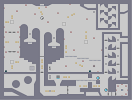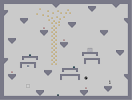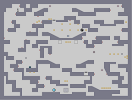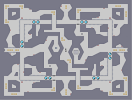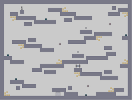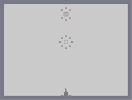Sometimes I don't wake up from my dreams. your guardian 没有 Hero To Save Them patch run (lowlife remix) Lift Yr. Skinny Fists Like Antennas To H Fuck You

Pages: (0)

### Hehe

It's actually a pretty damn good N-art.

### Everything after the I <3 U

(btw cool lettering on that)

so the chin and the uniform ^^
Oh COME ON! This is silly, how could this get a nomination for n-art of the year?!

Ah well, I guess it is a half nomination for me.

But still, I have done sooooooo much better.

^^"

### collab

Yes, i want to make a collab with you ;D

### boom!

IBAN STAR WARS
HOLY **** THAT IS AWESOME

Geez I need to watch at least a few old start trek episodes :P

Surprised that the N-art loaded in the thumb though, and it looks really good. I like how the uniform came out.

### Cool, love star wars

It's hard to see the details w/o full-size thumbnails, but I'm sure this looks better close up.

### if I'd know who is he

I would say something else..
but for now I'll say 5/5 ..me like wery mutch :)

XD

### YOU

DID THE SHIRT?
OR THE CHIN?
Hmmm, it really needs colouring in. Ah well.

?
Fastpostwin!